# Greatest common divisor

(diff) ← Older revision | Latest revision (diff) | Newer revision → (diff)

The largest of the common divisors of a set of integers or, in particular, of natural numbers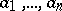. The greatest common divisor of a set of numbers not all of which are zero always exists. The greatest common divisor ofis usually denoted by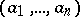.

Properties of the greatest common divisor are:

1) The greatest common divisor ofis divisible by any common divisor of these numbers.

2).

3) Ifare expressed aswhereare distinct primes,,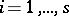, and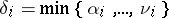, then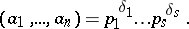The greatest common divisor of two natural numbers can be determined by the Euclidean algorithm. The number of steps necessary to do this is bounded from above by five times the number of digits in the smaller of the two numbers (in decimal notation).

The greatest common divisor of elements of an integral domain is defined as the common divisor of these elements that is divisible by any other common divisor. Thus, the greatest common divisor of two polynomials over a given field is the common divisor that is divisible by any other common divisor of the polynomials. If the greatest common divisor of two elements of an integral domain exists, it is unique up to multiplication by an invertible element. The greatest common divisor of two ideals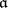andin a ring is the ideal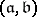generated by the union of the setsand(see Factorial ring).

How to Cite This Entry:
Greatest common divisor. Encyclopedia of Mathematics. URL: http://encyclopediaofmath.org/index.php?title=Greatest_common_divisor&oldid=17623
This article was adapted from an original article by A.A. BukhshtabV.I. Nechaev (originator), which appeared in Encyclopedia of Mathematics - ISBN 1402006098. See original article